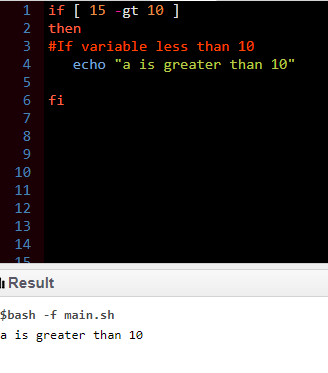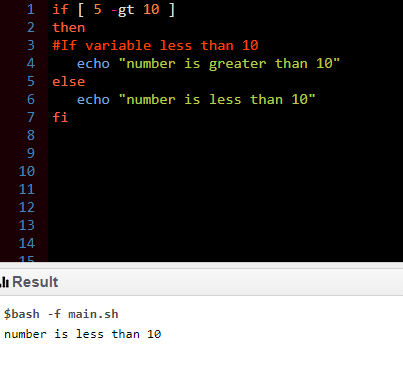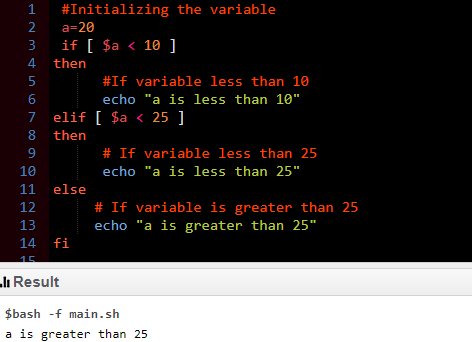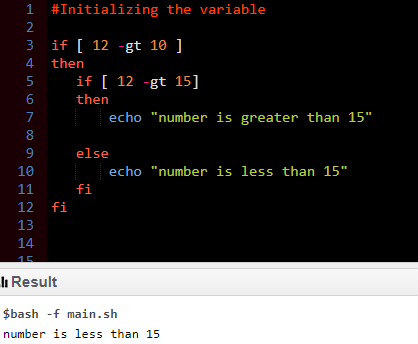GeeksforGeeks App
Open AppBrowser
Continue

# Bash Scripting – Else If Statement

In this article, we will discuss how to write a bash script for the Else If statement.

Conditional statements: The statements that perform specific functions based on certain conditions are called conditional statements. In bash scripting, we have several conditional statements like IF, IF-ELSE, IF-ELSE-IF, etc. Every statement has its way of working and according to the need, we use them.

## IF Statement

This statement is used when there is a need to check only conditions. If the condition founds to be true then the statement was written inside the if block will get executed.

Syntax:

```if (condition)
then
statement
fi```

Code:

```if [ 15 -gt 10 ]
then
# If variable less than 10
echo "a is greater than 10"

fi ```

This program will check the condition, whether 15 is greater than 10 or not. If 15 is greater than 10, the statement written inside the IF block will get printed on the screen.

Output:

`a is greater than 10`## IF-ELSE statement

As seen in the If statement, If the condition is true, the IF statement block gets executed but if the condition is false nothing is returned or executed. If we want the program to perform certain action after the IF statement condition is false, we use the ELSE statement after the If statement.

Syntax:

```if [condition ]
then
If statement
else
ELSE statement
fi ```
• If the condition is true: the IF statement will get executed.
• If the condition is false: the ELSE statement will get executed.

Code:

```if [ 5 -gt 10 ]
then
# If variable less than 10
echo "number is greater than 10"
else
echo "number is less than 10"
fi ```

Output:

`number is less than 10`## ELIF (ELSE IF) statement

ELIF is the keyword used for the ELSE IF statement in bash scripting. If in a loop if more than two conditions exist which can not be solved only by using IF-ELSE statement then ELIF is used. Multiple ELIF conditions can be defined inside one if-else loop.

ELIF syntax:

```if [ condition1 ]
then
statement1
elif [ condition2 ]
then
statement2
elif [condition3 ]
then
statement3
else
statement_n
fi```

Code:

```#!/bin/bash
# Initializing the variable
a=20
if [ \$a < 10 ]
then
# If variable less than 10
echo "a is less than 10"
elif [ \$a < 25 ]
then
# If variable less than 25
echo "a is less than 25"
else
# If variable is greater than 25
echo "a is greater than 25"
fi ```

Output:

`a is greater than 25`## NESTED statements

If one or more than one conditional statement is written inside another statement, this is called nested statements like IF statements inside another IF statement.

Syntax (Nested IF):

```If [condition]
then
if [condition_2]
then
statement_1

fi
fi```

Example:

```#!/bin/bash
#Initializing the variable

if [ 12 -gt 10 ]
then
if [ 12 -gt 15]
then
echo "number is greater than 15"

else
echo "number is less than 15"
fi
fi ```

Output:

`number is less than 15`My Personal Notes arrow_drop_up